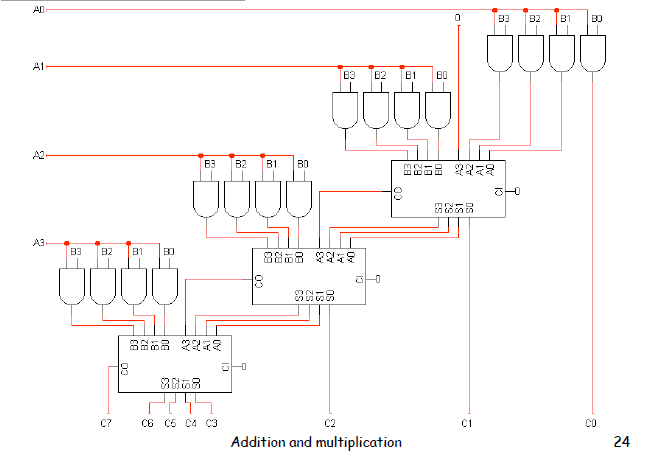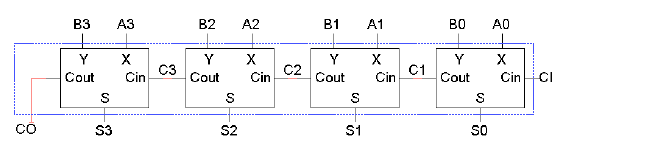Homework Statement

Build a circuit that either adds or multiplies two 4-bit numbers based on a control input C(C is 1 add, C is zero multiply). Numbers are positive and negative so use two's complement. You can use muxes, Full Adder Circuits, and logic gates

Homework Equations

Multiplier circuit=The Attempt at a Solution

So, I have built both the Full Adder(for the addition part) and the multiplier. However, I dont know how to use the muxes to get the input switching if control is 0 and 1. Since the mux only has one output, I dont understand how to connect the two circuits together. Any help is much appreciated!

mfb
Mentor
The mux output is the final result - one bit of the addition or multiplication depending on the state of C. Where do you expect more outputs? You'll need one mux per bit (or a mux block that can handle multiple bits in parallel).

Can you please show how we can use the mux to let the user choose between adder or multiplayer ?

mfb
Mentor
This thread is half a year old.
The multiplexer looks like every other multiplexer - for each bit, choose either input A or input B as output, depending on a control bit. Do that for all 4 bits.

•